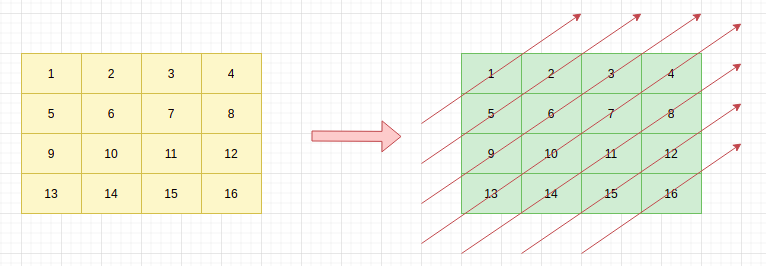New update is available. Click here to update.

# Print Diagonal

Posted: 30 Nov, 2020
Difficulty: Moderate

## PROBLEM STATEMENT

#### Example:#### Following will be the output of the above matrix:

``````1
5 2
9 6 3
13 10 7 4
14 11 8
15 12
16
``````
##### Input Format:
``````The first line contains an Integer 'T' which denotes the number of test cases or queries to be run. Then the test cases follow.

The first line of each test case contains two space-separated integers ‘N’ and ‘M’ denoting the number of rows and columns of the matrix respectively.

N’ lines follow. Each of the next ‘N’ lines contains ‘M’ space-separated integers separated by space.
``````
##### Output Format:
``````For each test case, return a 2D vector containing all elements of the matrix in a diagonal fashion.

The output of each test case should be printed in a separate line.
``````
##### Note:
``````You are not required to print anything, it has already been taken care of. Just implement the function.
``````
##### Constraints :
``````1 <= T <= 10
1 <= N <= 100
1 <= M <= 100
1 <= mat[i][j] <= 100

Time Limit : 1 sec.
``````